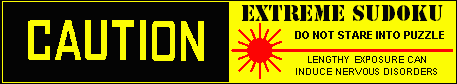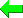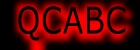Print Version
Solvers
Puzzles
Latest Apps
Str8ts
Other

## Discussion...

Post an idea here...
Your Name or Handle

Your comment, idea or question

 Please enter theletters you see:Remember me

Please keep your comments relevant to this puzzle.
Email addresses are never displayed, but they are required to confirm your comments. To state your solve time use the smaller form at the top right of this page." & vbNewLine & _ Line breaks and paragraphs are automatically converted ? no need to use <p> or <br> tags.

## ... by: Vinny & Manny

Ah, yes, we see now what you mean. You referred - we now assume - to the 2 not in Box 7 (which has to be in Row 7) but in Col 9 (that goes into Row 1). No problem! Our approach is that we have a sort of Fast Searcher Dog (techie term) that we let loose (he's marvellous!) with one or more Areas (boxes, rows, or columns) to sniff around, so we have to give him the set of 6 values, or 5, or maybe less) to be tried out in whatever Area, and, as the numbers in that set of 6 or whatever get entered, other values of that set get 'tied' to specific cell locations - all good. Then, as we're very thorough, we find the winning combination. Guessing has a connotation of being blindfolded, but, no, we'er sort of finding a fairly visible object in a house with a few different rooms in it - it's not a needle in a haystack. But you grabbed 3 numbers & solved it, well done.
Regards
Vinny & Manny

## ... by: Neil

Hi Vinny and Manny,

I think that you have misunderstood my initial comment. I said the 1 and 3 in Box 7, together with the 2 in Column 9 was sufficient to solve this puzzle. You showed 6 numbers to be entered in box 7, and 5 numbers in column 9. My comment about the 2 was for column 9.

In summary you used 11 guesses, I used 3 numbers.

Best regards

Neil
~

## ... by: Vinny & Manny

Hi, Neil,
We're interested in your method, you must elaborate it some time. Now, we notice that the 2 is given, in Row 9, but in Box 8, not in Box 7. So in Box 7, the 2 goes in row 7 or else row 8 - you said Row 9, maybe a typo? Now, we were perusing the various [initially] valid permutations of the 6 missing numbers in Box 7, which were nos. 1 to 6 [789 already given], and, upon trial of the permutation 213564 (left to right, rows 7 to 9), we effectively obliged the 1 & 3 to go where they went after placing the 2. But, hey, we got there!
V & M

## ... by: Neil

Yes, Vinny & Manny - the 2 does go into row 7 and col 1, but you don't need to put it there, as it's forced in by the 1 and 3.

Best regards

Neil
~

## ... by: Vinny & Manny

Hi, Neil,
I think you're right about the 1 & 3 but, in the solution, the 2 goes into row 7 in Col 1, does it not? NOw we were working through permutations of the possible 6 numbers to go into Box 7, and I see that once the 2 is placed, in Row 7 (Col 1), the 1 & 3 become a pair for r7c2 and r8c1, and the 45 become a pair in Row 9, Cols 1 & 3.
Pemutations! No getting round them! :-)
Vinny & Manny

## ... by: Paolo Calaresu

Basics J5=7;J7=8 and G1≠5.
After basics with only basics strategies
If A5=1=> B7≠1;B9≠4;C1≠23;C2≠3=>solution.

## ... by: ghfick

Thanks very much, Cenoman!

Just slightly more than a 'simple' AIC.
I need to study Steve K's blog as well.

Best Gordon

## ... by: Cenoman

Hi Gordon,
The name "Almost pattern x" is widely used on sudoku forums. I have no reference specific to "Almost Skyscraper", I learnt using almost patterns while reading solutions of other players.
You could read Steve K's blog page on "Almost finned swordfish example" here:
sudoku.com.au/sudokutips.aspx?Go=T13-1-1991
Very old stuff !
In the actual puzzle, "almost" means that there are additional candidates 4, beside the usual four candidates of a skyscraper, preventing the strong link 4C8=4C1 to exist, namely 4C56.
Through the chain (48-9)C56 = C4 - J4 = (9-4)J8, 4C56 is conflicting with 4J8 (the target of the skyscraper)
Therefore, in English:
WHETHER 4C56 OR skyscraper (4)C8=C1-G1=G9; in both cases J8<>4
It works like a finned fish. 4C56 would be called a "remote fin" (need of a chain)

Another presentation of the elimination is:
||(4)C8
||(4)C1 - G1 = G9
||(48-9)C56 = C4 - J4 = (9)J8
=> -4 J8
where the first two chains are a "kraken" presentation of the skyscraper.

In my chain (1)F8 = C8 - ^C4 = DF4* - E56 = E12** - DF3 = AB3 =>-1F56*, -1F2**, -1C2^
the characters *, **, ^ are used to point to the subchains used for each elimination.
(1)F8 = C8 - C4 = DF4 => -1F56
(1)F8 = C8 - C4 = DF4 - E56 = E12 => -1F2
(1)C4 = DF4 - E56 = E12 - DF3 = AB3 => -1C2
Regards, Cenoman

## ... by: numpl_npm

J1=4, J3=4 or J8=4 --> J2=6
A3=1, B3=1, D3=1 or F3=1 --> G9=4
D8=1, D8=2 or D8=9 --> A6=5
The rest can be solved.

## ... by: ghfick

Hi Cenoman,

I am not familiar with an 'Almost Skyscraper'. Could you point us to a link that explains its logic and origins? Or better, let us about it here?
Also can you explain your use of *, ** and ^ in your chains?

Best
Gordon

## ... by: Neil

Hi Vinny and Manny,

In your solution, you only need the 1 and 3 in Box 7, and then the 2 in row 9, col 1.

Best regards,

Neil
~

## ... by: Cenoman

This puzzle has a Jexocet pattern, detected by Andrew's solver, but not completely used.
Following David P Bird's JExocet Compendium:
1. JExocet (1234)JE2: C1,B4,A8
Eliminations (with #n, elimination rule number inDPB's compendium:
-9B4 (non-base digit in target cell; #3)
-1B4 (1 can't be true in the mirror node A79; #6)
-45A9 (A7=6 =>A9 restricted to base digits in B4; #7)
-9B56 (locked 6@B56; #9)
Basics: A6=5, C79=57, -9C8

From there, the puzzle is solved with three AICs & Basics
2. Almost Skyscraper [(4)C8*=C1-G1=G9] = (48-9)C56 = C4 - J4 = (9)J8 => -4 J8
Basics: G9=4, G7=5=C9, C7=7, J48=39, J2=6, ABH9=239, E9=6, DF1=68, J1=5, J3=4=C1, A8=4, B56=46, -2H1
3. (3=2)B4 - (2=1)A5 - (1=8)G5 - C5 = (8-3)C6 = (3)GH6 =>-3J4
Basics: J4=9, J8=3=E7, C56=89, -9F8, D8=9, -3G2
4. (1)F8=C8 - ^C4 = DF4* - E56 = E12** - DF3 = AB3 =>-1F56*, -1F2**, -1C2^; Basics to the end.

## ... by: Vinny & Manny

Spread 213564 across the empty squares in box 7 (from top left to lower right). Work (hard!) with that for a bit. Enter 23879, top to bottom, along the [at that point] empty cells of col 9. That should lead to the solution.
Vinny & Manny

## ... by: Frans Goosens

With trial and error

Combination D3=125 and H7=239

************************************************************
D3=1 H7=2 No solution, Fixed

Calculate 2 digits cells

D7=7 No solution, Undo calculation
D7=9 Wrong, Undo calculation
D7=7 No solution, Fixed
D8=2 No solution, Undo calculation
D8=9 No solution, Undo calculation
G2=2 No solution, Undo calculation
G2=3 Wrong, Undo calculation
G2=2 No solution, Fixed
D8=2 No solution, Undo calculation
D8=9 Wrong, Undo calculation
D8=2 No solution, Fixed
A5=2 Wrong, Undo calculation
A5=4 Wrong, Undo calculation

All reset to initial position

************************************************************
D3=1 H7=3 No solution, Fixed

Calculate 2 digits cells

D8=2 Wrong, Undo calculation
D8=9 Wrong, Undo calculation

All reset to initial position

************************************************************
D3=1 H7=9 No solution, Fixed

Calculate 2 digits cells

D4=2 Wrong, Undo calculation
D4=5 No solution, Fixed
D6=6 Wrong, Undo calculation
D6=9 Wrong, Undo calculation

All reset to initial position

************************************************************
D3=2 H7=2 No solution, Fixed

Calculate 2 digits cells

D8=1 No solution, Undo calculation
D8=9 No solution, Undo calculation
G5=1 No solution, Undo calculation
G5=8 No solution, Undo calculation
G7=3 Wrong, Undo calculation
G7=5 No solution, Fixed
G9=3 Wrong, Undo calculation
G9=4 ( I3=4 ) Solved,

#313----------------------------Solution
980 700 600-------------983 715 642
750 000 080-------------751 246 983
006 000 000-------------426 398 715

040 030 000-------------642 537 198
007 800 050-------------197 824 356
000 000 400-------------835 169 427

009 600 070-------------219 683 574
078 450 060-------------378 451 269
000 002 001-------------564 972 831

Total solving time is: 441 sec.

## Archive

Each week a new 'unsolvable' will be published and the previous will be accessible here from this archive section. If you like very tough puzzles, these are for you.
Share and Enjoy

Page created on 03-May-2011, last modified on 03-May-2011.
All puzzles on this site are trademarked and copyright
and cannot be reproduced without permission.
Copyright Syndicated Puzzles Inc, 2011-2015